# Difference between revisions of "Probability space"

probability field

$\newcommand{\Om}{\Omega} \newcommand{\A}{\mathcal A} \newcommand{\P}{\mathbb P}$ A triple $(\Om,\A,\P)$ consisting of a non-empty set $\Om$, a class $\A$ of subsets of $\Om$ which is a σ-algebra (i.e. is closed with respect to the set-theoretic operations executed a countable number of times) and a probability measure $\P$ on $\A$. The concept of a probability space is due to A.N. Kolmogorov . The points of $\Om$ are said to be elementary events, while the set $\Om$ itself is referred to as the space of elementary events or the sample space. The subsets of $\Om$ belonging to $\A$ are (random) events. The study of probability spaces is often restricted to the study of complete probability spaces, i.e. spaces which satisfy the requirement $B\in\A$, $A\subset B$, $\P(B)=0$ implies $A\in\A$. If $(\Om,\A,\P)$ is an arbitrary probability space, the class of sets of the type $A\cup N$, where $A\in\A$ and $N\subset M$, for some $M\in\A$ with $\P(M)=0$, forms a σ-algebra $\overline{\A}$, while the function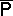on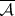defined by the formula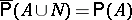is a probability measure on. The space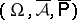is complete and is said to be the completion of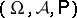. Usually one may restrict attention perfect probability spaces, i.e. spaces such that for any real-measurable functionand any seton the real line for which, there exists a Borel setsuch that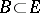and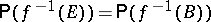. Certain "pathological" effects (connected with the existence of conditional probabilities, the definition of independent random variables, etc.), which occur in the general scheme, cannot occur in perfect probability spaces. The problem of the existence of probability spaces satisfying some given special requirements is not trivial in many cases. One result of this type is the fundamental Kolmogorov consistency theorem: Let to each ordered-tuple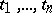of elements of a setcorrespond a probability measureon the Borel sets of the Euclidean spaceand let the following consistency conditions be satisfied:

1)for all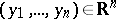, whereandis an arbitrary rearrangement of the numbers;

2).

Then there exists a probability measure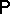on the smallest-algebraof subsets of the product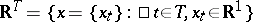with respect to which all the coordinate functions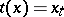are measurable, such that for any finite subsetofand for any-dimensional Borel setthe following equation is true: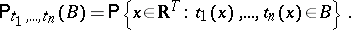How to Cite This Entry:
Probability space. Encyclopedia of Mathematics. URL: http://encyclopediaofmath.org/index.php?title=Probability_space&oldid=19753
This article was adapted from an original article by V.V. Sazonov (originator), which appeared in Encyclopedia of Mathematics - ISBN 1402006098. See original article